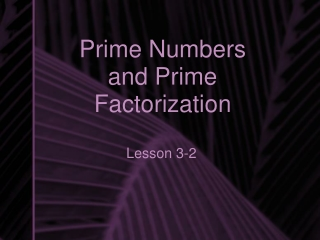Download PresentationPrime Numbers and Prime Factorization

# Prime Numbers and Prime Factorization

Télécharger la présentation## Prime Numbers and Prime Factorization

- - - - - - - - - - - - - - - - - - - - - - - - - - - E N D - - - - - - - - - - - - - - - - - - - - - - - - - - -
##### Presentation Transcript

1. Prime Numbers and Prime Factorization Lesson 3-2

2. Factors • Factors are the numbers you multiply together to get a product. • For example, the product 24 has several factors. • 24 = 1 x 24 • 24 = 2 x 12 • 24 = 3 x 8 • 24 = 4 x 6 • SO, the factors are 1, 2, 3, 4, 6, 8, 12, 24

3. Finding Factors • Start with 1 times the number. • Try 2, 3, 4, etc. • When you repeat your factors, cross out the repeat - you’re done at this point. • If you get doubles (such as 4 x 4), then you’re done. Repeats or doubles let you know you’re done.

4. What are the factors of 16? 1 x 16 2 x 8 3 x ?? 3 is not a factor, so cross it out 4 x 4 doubles = done The factors of 16 are 1,2,4,8,16

5. What are the factors of 18? 1 x 18 The factors are 1,2,3,6,9,18 2 x 9 3 x 6 4 x ?? 5 x ?? 6 x 3 Repeat! Cross it out! We’re done!

6. What are the factors of 7? The only factors of 7 are 1,7 1 x 7 2 x ?? 3 x ?? 4 x ?? 5 x ?? 6 x ?? 7 x 1 This works, but it is a repeat. We are done.

7. Prime numbers are numbers that only have two factors: one, and the number itself. EXAMPLES: 3, 5, 7, 11, 31 Composite numbers have more than two factors. EXAMPLES: 6, 15, 18, 30, 100 Prime and Composite Numbers

8. A Product of Primes • Every composite number can be expressed as a product of prime numbers. • This is called prime factorization.

9. Example • 15 is a composite number. • It can be expressed as a product of primes: 3 x 5

10. To find the prime factorization: • Divide the number by the first prime number possible. • Circle the prime number, and continue with the other factor. • Divide the new factor by a prime number. • Continue this process until the only numbers you have left are prime numbers.

11. Remember the Prime Number List: • 2, 3, 5, 7, 11, 13, 17, 19, 23, 29, 31, 37, 41, 43, 47, 53, 59, 61, 67, 71, 73, 79, 83, 89, 97…

12. Example: Prime Factorization of 100. 100 100 ÷ 2 = 50. Two is the first prime number that goes into 100. Now we deal with the 50. Divide it by 2 to get the next factors. 2 is a prime number, so we are done with it. 2 X 50 25 is not divisible by the first prime, 2. The next prime, 3, does not work either. We must divide by 5 to get a factor. 2 X 25 5 x 5 Both numbers are prime, leaving us with all primes.

13. What’s the Answer? • Now, we just list our factors with multiplication signs between them. Use the circled prime numbers. • 2 x 2 x 5 x 5 • We have listed 100 as a product of prime numbers.

14. Exponent Form • We have just listed our prime factorization for 100 as being 2 x 2 x 5 x 5. This is repeated multiplication. Repeated multiplication can be expressed with exponents. • Our prime numbers are our bases. The number of times the prime number is written is the exponent. • 2 x 2 can be expressed in exponent form: 22 • 5 x 5 can be expressed as 52 • Put it together, and 2 x 2 x 5 x 5 is more simply put as 22 x 52

15. Another Example 420 2 x 210 2 x 105 22 x 3 x 5 x 7 3 x 35 5 x 7